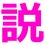# AM-GM Struggle! (1)

I have a problem, which is not really sure for me. Absolutely, it is about the inequalities!

For $$a,b,c \in \mathbb{R^{+}}$$, if $$abc=1$$, then proof that $a^2+b^2+c^2 \geq a+b+c$

My proof is like this:

Since $abc=1$, then according to AM-GM, we provide $\frac{a+b+c}{3} \geq \sqrt{abc}$ $\Rightarrow a+b+c \geq 3$

Consider these: $(2a-1)^2 + (2b-1)^2 + (2c-1)^2 \geq 0$

by QM-AM, we provide $\sqrt{\frac{(2a-1)^2 + (2b-1)^2 + (2c-1)^2}{3}} \geq \frac{(2a-1)+(2b-1) + (2c-1)}{3} = \frac{2(a+b+c)-3}{3}$

Inserting the first AM-GM inequality, we get $\sqrt{\frac{(2a-1)^2 + (2b-1)^2 + (2c-1)^2}{3}} \geq \frac{2(a+b+c)-3}{3}$ $\geq \frac{2(3)-3}{3} = 1$

Then, solve it. $\sqrt{\frac{(2a-1)^2 + (2b-1)^2 + (2c-1)^2}{3}} \geq 1$ $\Rightarrow (2a-1)^2+(2b-1)^2+(2c-1)^2 \geq 3$ $\Rightarrow 4(a^2+b^2+c^2) - 4(a+b+c) +3 \geq 3$ $\Rightarrow 4(a^2+b^2+c^2)-4(a+b+c) \geq 0$ $\Rightarrow a^2 +b^2 +c^2 \geq a+b+c$ $\blacksquare$

I had been inspired by filling the perfect squares so it provides $(2a-1)^2 +(2b-1)^2 + (2c-1)^2 \geq 3$ Is that valid for the proof? Any comments will be appreciated.Note by Figel Ilham
6 years ago

This discussion board is a place to discuss our Daily Challenges and the math and science related to those challenges. Explanations are more than just a solution — they should explain the steps and thinking strategies that you used to obtain the solution. Comments should further the discussion of math and science.

When posting on Brilliant:

• Use the emojis to react to an explanation, whether you're congratulating a job well done , or just really confused .
• Ask specific questions about the challenge or the steps in somebody's explanation. Well-posed questions can add a lot to the discussion, but posting "I don't understand!" doesn't help anyone.
• Try to contribute something new to the discussion, whether it is an extension, generalization or other idea related to the challenge.

MarkdownAppears as
*italics* or _italics_ italics
**bold** or __bold__ bold
- bulleted- list
• bulleted
• list
1. numbered2. list
1. numbered
2. list
Note: you must add a full line of space before and after lists for them to show up correctly
paragraph 1paragraph 2

paragraph 1

paragraph 2

[example link](https://brilliant.org)example link
> This is a quote
This is a quote
    # I indented these lines
# 4 spaces, and now they show
# up as a code block.

print "hello world"
# I indented these lines
# 4 spaces, and now they show
# up as a code block.

print "hello world"
MathAppears as
Remember to wrap math in $$ ... $$ or $ ... $ to ensure proper formatting.
2 \times 3 $2 \times 3$
2^{34} $2^{34}$
a_{i-1} $a_{i-1}$
\frac{2}{3} $\frac{2}{3}$
\sqrt{2} $\sqrt{2}$
\sum_{i=1}^3 $\sum_{i=1}^3$
\sin \theta $\sin \theta$
\boxed{123} $\boxed{123}$

Sort by:

You can simply use cauchy schwarz inequality

- 6 years ago

I got it. But, I want to learn advance for AM-GM topic.

- 6 years ago